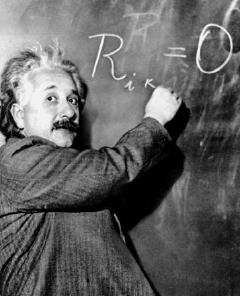NextPrevious

# Why is the equation E = mc2 historically significant?

The famous equation was put forth in 1905 by German-born physicist and Nobel laureate (1921) Albert Einstein (1879–1955) and became important to history largely because it, along with the quantum theory (developed by Max Planck in 1900) laid the foundation for nuclear energy. It is part of Einstein’s theory of relativity (1905, 1916).

In the formula, E is energy, m is mass, and c-squared is a constant factor equal to the speed of light squared. The equation illustrates the relationship between, and exchangeability of, energy and matter. In the 1930s, when scientists discovered a way to split atoms (the minute particles of which elements are made), they learned that the subatomic particles that were created have a total mass less than the mass of the original atom. In other words, when the atom was split, part of the mass of the atom had been changed into subatomic particles but some of it had been converted into energy. Using Einstein’s formula E = mc2, the scientists calculated how much energy was produced by splitting an atom. This atom-splitting method for creating energy is the basis for nuclear energy. The term nuclear refers to the atomic process, which uses the nucleus or central portion of an atom to release energy. Today, we live in the nuclear age, where power and weapons are produced using the atomic process for creating energy.Albert Einstein writes an equation for the density of the Milky Way in 1931. Einstein is best known for his theory of relativity.
Close

This is a web preview of the "The Handy History Answer Book" app. Many features only work on your mobile device. If you like what you see, we hope you will consider buying. Get the App